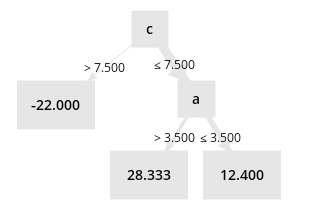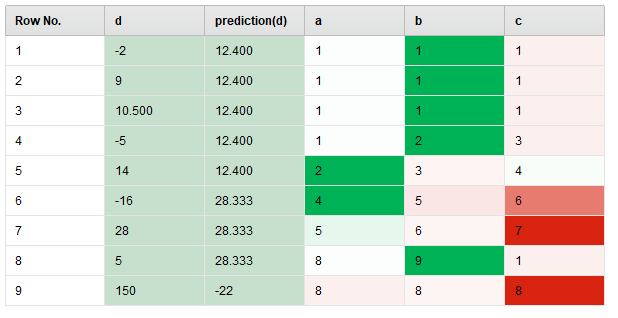# Explain Predictions interpretation for regression

Member Posts: 50Guru
edited March 2020 in Help
I have been experimenting with visualization of the results of the Explain Predictions operator. My objective is to evaluate if it could be used as a tool to investigate cases where the model was wrong, by finding which variables contributed the most to the error. The error is defined as the difference between the actual value and the predicted value. However I'm not sure how to interpret the values returned by Explain Predictions.

To help understand, I built a simple example with a simple data set that trains a decision tree and tries to explain its prediction. But I don't understand in this example how it decides which attributes "support" the prediction and which ones "contradict" it.Output of the Explain Predictions "vis" port:Examples I'm trying to understand:
* In example 7 and 8, why is column "c" considered to be contradicting the prediction? If we follow the path in the decision tree for this example, we get to the value of the prediction (28.333 for example 7, -22 for column 9), so shouldn't this support the prediction?

* In the first 4 examples, why is b considered to be supporting the prediction, but not a or c?

Also, I noticed that the "imp" output port doesn't take in consideration the "maximal explaining attributes" parameter (it always returns all attributes for all examples). Is there a way to limit it? As a workaround I built a process that uses the outputs of the "exa" port and parses the string values of the "Support Prediction" and "Contradict Prediction" columns to get this information but limited to the top supporting/contradicting attributes for each example. Is there a simpler solution I didn't see?

Tagged:

• Member Posts: 50Guru